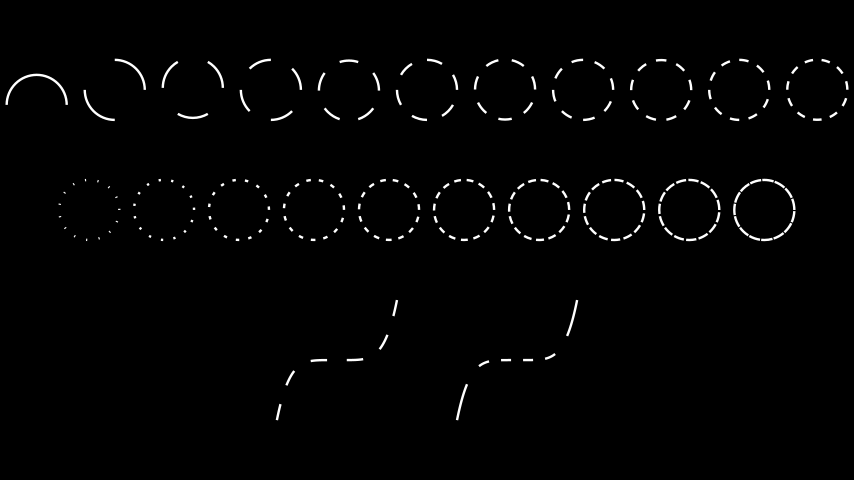# DashedVMobject#

Qualified name: `manim.mobject.types.vectorized\_mobject.DashedVMobject`

class DashedVMobject(vmobject, num_dashes=15, dashed_ratio=0.5, dash_offset=0, color='#FFFFFF', equal_lengths=True, **kwargs)[source]#

Bases: `VMobject`

A `VMobject` composed of dashes instead of lines.

Parameters
• vmobject – The object that will get dashed

• num_dashes – Number of dashes to add.

• dashed_ratio – Ratio of dash to empty space.

• dash_offset – Shifts the starting point of dashes along the path. Value 1 shifts by one full dash length.

• equal_lengths – If `True`, dashes will be (approximately) equally long. If `False`, dashes will be split evenly in the curve’s input t variable (legacy behavior).

Examples

Example: DashedVMobjectExample```from manim import *

class DashedVMobjectExample(Scene):
def construct(self):
r = 0.5

top_row = VGroup()  # Increasing num_dashes
for dashes in range(1, 12):

middle_row = VGroup()  # Increasing dashed_ratio
for ratio in np.arange(1 / 11, 1, 1 / 11):
circ = DashedVMobject(
)

func1 = FunctionGraph(lambda t: t**5,[-1,1],color=WHITE)
func_even = DashedVMobject(func1,num_dashes=6,equal_lengths=True)
func_stretched = DashedVMobject(func1, num_dashes=6, equal_lengths=False)
bottom_row = VGroup(func_even,func_stretched)

top_row.arrange(buff=0.3)
middle_row.arrange()
bottom_row.arrange(buff=1)
everything = VGroup(top_row, middle_row, bottom_row).arrange(DOWN, buff=1)
 `animate` Used to animate the application of any method of `self`. `animation_overrides` `color` `depth` The depth of the mobject. `fill_color` If there are multiple colors (for gradient) this returns the first one `height` The height of the mobject. `n_points_per_curve` `sheen_factor` `stroke_color` `width` The width of the mobject.